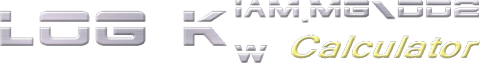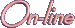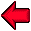The method used to predict both Log kwIAM.MG and Log kwIAM.DD2 is based on these equations:

Log kwIAM.MG = -0.1405 (± 0.1282) + 0.4401 (± 0.0297) miLogP + 0.0536 (± 0.0057)
Heavy_Atoms - 0.0833 (± 0.0201) HLBM - 0.0435 (± 0.0144) Rotatable_bonds

n = 204 r2 = 0.81 q2 = 0.80 SE = 0.438 F4,199 = 213.92 P < 1.0 10-8 PC = 39.403

Log kwIAM.DD2 = -2.3989 (± 0.2812) + 0.4936 (± 0.0379) miLogP + 0.4354 (± 0.0470)
Volume_Diameter - 0.0640 (± 0.0226) HLBPSA - 0.0497(± 0.0173) Rotatable_bond
s

n = 160 r2 = 0.85 q2 = 0.84 SE = 0.459 F4,155 = 212.94 P < 1.0 10-8 PC = 33.974

where:

miLogP is the log P predicted by the method developed by Molinspiration Chemoinformatics, Hravy_Atoms is the number of heavy atoms, HLBPSA is the hydrophilic-lipophilic balance calculated with the PSA method (see below), HLBM is the mean of the hydrophilic-lipophilic balance values predicted with three different methods (Griffin, Davies and PSA), Rotable_bonds is the number of rotatable dhiedral angles and Volume_diameter is the diameter length of a hypothetical sphere with the same volume of the molecule.

HLBPSA is defined by the following expression:

HLBPSA = 20 * PSA / Surface

where PSA is the polar surface area and Surface is the total surface.

The complete discussion of the method is available in Eur J Pharm Sci. 2016 Dec 2. pii: S0928-0987(16)30519-X (doi: 10.1016/j.ejps.2016.11.026).

These equations were implemented in this Web service that can predict Log kw values following these steps:

1. The service connects itself to PubChem to search and download the 3D structure of the molecule specified by the user. PUG REST APIs are used to interface the service to PubChem.
2. VEGA command line calculates the molecular descriptors (HLBs, number of rotatable dihedrals, volume  diameter) and  converts the structure to SMILES.
3. The SMILES string is sent to Molinspiration Web service to retrieve miLogP. RESTful interface is used to get the property.
4. Each property value is checked if it's in the range of values of the training set used to derive the QSAR models. If the property is outside of the prediction domain, a warning message is shown.
5. Finally, Log kw values are calculated and shown in the page.Return to the Log kwIAM.MG/DD2 service